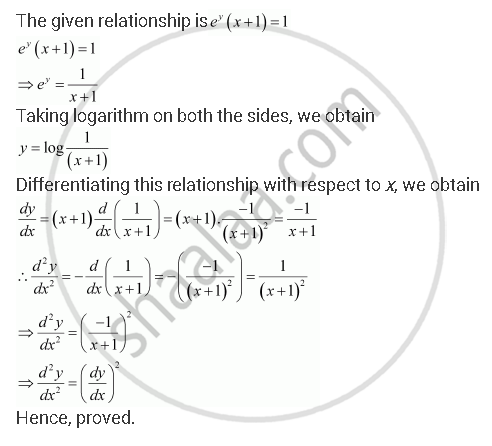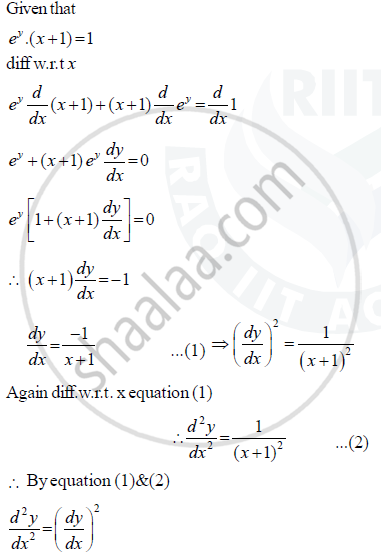# If Ey (X + 1) = 1, Show That (D^2y)/(Dx^2) =((Dy)/(Dx))^2 - Mathematics

If ey (x + 1) = 1, show that  (d^2y)/(dx^2) =((dy)/(dx))^2

#### Solution 1#### Solution 2Concept: Second Order Derivative
Is there an error in this question or solution?
Chapter 5: Continuity and Differentiability - Exercise 5.7 [Page 184]

#### APPEARS IN

NCERT Class 12 Maths
Chapter 5 Continuity and Differentiability
Exercise 5.7 | Q 16 | Page 184

Share